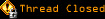# Topic awaiting preservation: If there is no BIGGEST number, is there a SMALLEST? (Page 1 of 1)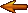loarevalo
Obsessive-Compulsive (I) Inmate

From:
Insane since: Jul 2005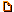posted 07-30-2005 04:00

The problem is this:

Set Theory, and the whole of Mathematics, requires that there be no Absolute Infinity defined, no number that could be called "the last number" or the biggest. Yet we use an "smallest number" - the number zero.

If there is no largest number, no absolute infinity, no last number (and the consistency of mathematics rests on this premise), it is only intuitive for symmetry's sake to suggest that, likewise, there must not be a smallest number, zero.

Philosophically speaking, the ideas "one-half" and "two" are equivalent, though we differentiate them mathematically by the particular meaning attached to them, like we differentiate between "having two" and "not having two." Both "negative two" and "two" allude to the number "two," and both are equivalent in the sense that they are both that same "two," only in different contexts, thus acquiring their different meanings. Likewise "one-half" and "two" allude to the same number and are equivalent ideas in that sense - "one-half" could not exist without "two." It follows that if such an absolute infinity is not subject to determination or definition, so does not an absolute infinitesimal, which we have named zero.

Nonetheless, zero does exist, in the sense that infinitesimals do exist because infinite numbers do also. One minus one is still zero, if by zero we mean "some infinitesimal," if zero is defined as the number (or any number) that added to a finite number it is equivalent to the same finite quantity. Likewise, a characteristic of a transfinite number is that such a number when added to a finite number, results in another transfinite quantity (this suffices though we may also say 'it is the same transfinite number').

I have not found, and there may not be, a rigorous proof that zero does not exist in mathematics. But I can see how we would do so much better if we did not appeal to an absolute zero. Because we do, I suppose, is the current hinder of all versions of Analysis, because we have not yet defined within the system a true infinitesimal. Nonstandard Analysis's so called infinitesimals are not infinitesimals, but numbers smaller than the smallest reals - "thinner" than reals. A comprehensive system for infinitesimals would disallow the use of an absolute zero, as classic set theory disallows absolute collections.

Besides metaphysics, besides the nature or definition of zero, one point I want to make clear: the infinitesimal is defined under the notion and definition of infinity if we are to define the term 'infinitesimal' as 'infinitely small.' The true infinitesimal, added to a finite, results in that same finite - not an approximate finite. Thus far I have not learned of any system that defines true infinitesimals.

If for an infinite N: N + 1 = N
Then for an infinitesimal n: 1 + n = 1 or 1 + 1/N = 1

NSA's infinitesimal n: 1 + n ~ 1 or std(1+n) = 1
One plus n is infinitely close to one.

This insight may bring us to greater understanding of many mischeavous behaviours like division by zero, and the called indeterminate forms. If the absolute zero is not defined, division by the same is not defined (as it currently is); for poet's sake we may say that division by zero is the absolute infinity, but that also is undefined. Because by using zero we usually mean something other than the absolute zero (or not necesarily the absolute zero), zero divided by zero is undetermined not due to the operation or form, but to the undetermined nature of zero. As we mean many things (allude to many numbers) by the generic "infinity" so we mean various things by a simple "zero" - that could be a specific infinitesimal, an undetermined class of infinitesimals, or even the absolute zero.

Thank you for your attention. If you know of any reasoning that deals with these issues, please inform me of such.

O.A.

reisio
Paranoid (IV) Inmate

From: Florida
Insane since: Mar 2005posted 07-30-2005 04:46

Looks like the NSA's has a venereal disease.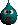I don't see a problem, but IANAM, nor do I think we need anything new to fuel our ridiculous technological cravings.

outcydr
Paranoid (IV) Inmate

From: out there
Insane since: Oct 2001posted 07-30-2005 07:20

it's no coincidence that zero is represented as
a circle with no set beginning or ending

tricky digit that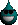WebShaman

From: Happy Hunting Grounds...
Insane since: Mar 2001posted 07-30-2005 12:57

Actually, zero is "in the middle" between positive and negative integers.

So no, there is no beginning and no end.

WarMage

From: Rochester, New York, USA
Insane since: May 2000posted 08-01-2005 21:13

In addition, zero is a pretty vacuous number, with lots of properties assigned to it by denfinition in the same fashion of those assigned to infinity.

But at the same time you are grasping at straws. You can not say that one of these things imply the other, you might like to think that, but it does not make it true.

You also say that the consistency of mathematics is based on the fact that you have an infinity. I have not seen this in my studies. When you setup a mathematical stystem you do not base it on an infinity, you base it on 0 then on 1 maybe you add in a -1 and you give the system the rule for addition. Have at it and through recursion you have an infinity. You do not base your system on infinity.

Dan @ Code Town

loarevalo
Obsessive-Compulsive (I) Inmate

From:
Insane since: Jul 2005posted 08-02-2005 06:12

Thanks for those responses. But I don't know if there are supporting the argument or not. If you are, please tell any suggestions as to how to proceed.

I didn't say that the consistency of math depends on the existence of infinity (though it does, how else could you have the set of all natural numbers, or the set of reals, etc..). I said that the consistency of math depends upon the notion that there is no LARGEST number, no Absolute Infinity, no number that we could say "it's the last one"

I am more of the opinion that we should base our number system on the number 1, and number 2, and build from there. Once we get to infinity (with methods like of Set Theory) we can then define infinitesimals, or zero. I think that we would a much better system.

O.A.

WarMage

From: Rochester, New York, USA
Insane since: May 2000posted 08-02-2005 14:18

Well... all of the sets of numbers that I work with on a regular basis are finite.

Z mod p where p is a very large prime number.

Dan @ Code Town

hyperbole
Paranoid (IV) Inmate

Insane since: Aug 2000posted 08-02-2005 18:18

I took a course in "Applied Math" many years ago in which we studied things like this. I remember that we were asked to prove that 1 is greater than 0 and I think we had to prove the existence of 0. "Proof by Contradiction" was the recommended method in both cases.

I would try a "Proof by Contradiction" technique to prove your theory.

I assume you know "Proof by Contradiction", but just in case you don't (or for someone else who may not be familiar with it)

1. You have a theorem

2. Assume the opposite of your theorem is true.

3. Prove that the opposite is false.

4. By contradiction your theorem must be true since the opposite is false.

n.b. There are some mathematicians who say that proof by contradiction is not a valid way of proving a theorem, because the opposite of a false assumption is not necessarily true.

.

-- not necessarily stoned... just beautiful.Raeubu
Obsessive-Compulsive (I) Inmate

From: Kennewick, WA
Insane since: Aug 2005posted 08-03-2005 12:44

Well, I'm definitely not a mathematician, but for the sake of controversy: You could say that 0 is the lack of a number, and because there can be an infinite number of places to the right of a decimal point, it would be impossible to find the smallest number without having the lack of a number. If that makes any sense?

Quidquid Latine Dictum Sit, Altum Viditur ~
Whatever is said in Latin sounds profound
-Ray

wrayal
Bipolar (III) Inmate

From: Cranleigh, Surrey, England
Insane since: May 2003posted 08-04-2005 13:46

"zero divided by zero is undetermined not due to the operation or form, but to the undetermined nature of zero."

Hmm...I'd contend that this is not quite true. In set theory, 0 is defined as the digit such that a+0=a. Hence, this gives a rigorous definition which, when applied with the other usual axioms, can build the number system.
Rather, 0 divided 0 is undefined, not due to the uncertain nature of 0, but due to the fact that there is no clear way to apply division in this situation, nor any other given situation where a divsion by zero is invloved. Perhaps I misuderstood, but I would suggest that 0 is absolute, i.e. not an 'infinitesimal value' as 1/infinity might indicate. (look at 1/-infinity. This would give an different value for 0. Infinitesiamlly different perhaps, but different nevertheless)

 To answer the actual question, I'd suggest as n->infinity 1/n->smallest number. Much as with the 'largest number', I'd suggest you cannot directly express the smallest number[/edit]

Wrayal(Edited by wrayal on 08-04-2005 13:48)

loarevalo
Obsessive-Compulsive (I) Inmate

From:
Insane since: Jul 2005posted 08-07-2005 01:08

The issue isn't that you can't express the largest number - it's that you can't even say it exists, for reason of contradiction.

O.A.

loarevalo
Obsessive-Compulsive (I) Inmate

From:
Insane since: Jul 2005posted 08-07-2005 01:18

Some of you seem confused about what I am arguing for, and what I rely on as "established" facts in mathematics. Because these "facts" may not be so widely known as I thought, I include a reference.

*FACTS:*

* There are many sizes of infinity. There is a marked difference between the potential infinity of Arithmetic and Calculus, and the actual infinities dealt in Set Theory:
http://en.wikipedia.org/wiki/Cardinal_number
http://en.wikipedia.org/wiki/Aleph_number

* The Absolute Infinity - Biggest number - set containing all sets does NOT exist. The very definition of it is inconsistent: To quote from the source, "there can be no end to the process of set formation, and thus no such thing as the totality of all sets, or the set hierarchy. Any such totality would itself have to be a set, thus lying somewhere within the hierarchy and thus failing to contain every set."
http://en.wikipedia.org/wiki/Absolute_infinite

* Infinity plus one is Infinity. That fact I think dwells on the obvious. Aleph_a +1 = Aleph_a (Example: Aleph_null +1 = Aleph_null):
http://mathworld.wolfram.com/Aleph-0.html

As we see, Infinity is very complex. Should we not also allow that complexity to infinitesimals?

I argue for the same that the FACTS establish for infinity; only, that instead of infinity, I apply them to the infinitesimals, like a mirror. All I am arguing for is symmetry.

- Since there are many sizes of infinity,
therefore there are many sizes (degrees) of infinitesimals
(Leibnez and Euler also argued this).

- Since INF + 1 = INF,
therefore Infinitesimal + 1 = 1

- Since Absolute Infinity does not exist
therefore Absolute Zero does not exist.

(I consider the terms "zero" and "infinity" equally vague. I also use the term "zero" and "infinitesimal" interchangeably. If you say "zero," I know you probably mean "Absolute zero")

O.A.

WebShaman

From: Happy Hunting Grounds...
Insane since: Mar 2001posted 08-07-2005 04:24

In other words, "zero" is in the middle of infinity., where -infinity and +infinity are =

They come full circle, and 0 is in the middle, as I said.

Slime

From: Massachusetts, USA
Insane since: Mar 2000posted 08-07-2005 04:53
quote:
As we see, Infinity is very complex. Should we not also allow that complexity to infinitesimals?

What we "allow" is irrelevant. The properties of infinity that you mentioned, as well as every other property of mathematics, are derived from simple rules. Beyond those basic ideas, philosophical thought is irrelevant - if you agree to them, you agree to everything that's been proven with them as a base.

quote:
I have not found, and there may not be, a rigorous proof that zero does not exist in mathematics

Such a proof won't be found, because zero is a product of the properties given to the set we call the real numbers. Its existence within this set can be proven. Now, if you don't like the properties of the real number system, you can come up with another system that has different properties and talk about that one instead. That's the nice thing about math - it's not about what we want or believe, it's more solid than that.

If you want to talk philosophically about the existance of zero, you can't link it to math very strongly. You could talk, for instance, about how much relevance the real number system has to real life, and say, maybe, that the existance of zero in the real numbers doesn't imply the possibility of nothingness in the real world.

You could discuss the set of numbers greater than zero, which does have similarities (near zero) to infinity. But zero itself, I'm afraid, does exist.wrayal
Bipolar (III) Inmate

From: Cranleigh, Surrey, England
Insane since: May 2003posted 08-07-2005 12:00

Perhaps a pedantic point, but how can 0 lie within the realm of real numbers? Technically 0 is a digit, not a number....Zynx
Obsessive-Compulsive (I) Inmate

From:
Insane since: Aug 2005posted 08-18-2005 00:55

No matter what "number" exists, you can always mathematically divide it by 2.

quote:
WebShaman said: Actually, zero is "in the middle" between positive and negative integers. So no, there is no beginning and no end.

Truth don't get any better than this.

loarevalo
Obsessive-Compulsive (I) Inmate

From:
Insane since: Jul 2005posted 08-19-2005 22:08

For an infinitesimal x, could we agree on this?:

1 + x = 1

If not, then for an infinite X, why do you agree on this?:

X + 1 = X

As I see it, these two should be equivalent, since the concept of the infinitesimal derives directly from the concept of infinity.

Adding a finite to an infinite does not increase the infinite,
so, adding an infinitesimal to a finite, does not increase the finite.

Is this not reasonable?

O.A.

Zynx
Nervous Wreck (II) Inmate

From:
Insane since: Aug 2005posted 08-26-2005 02:28
quote:
For an infinitesimal x, could we agree on this?: 1 + x = 1
If not, then for an infinite X, why do you agree on this?: X + 1 = X
As I see it, these two should be equivalent, since the concept of the
infinitesimal derives directly from the concept of infinity.
Adding a finite to an infinite does not increase the infinite,
so, adding an infinitesimal to a finite, does not increase the finite.
Is this not reasonable?
O.A.

Confusing concepts of mathematical theoretical extrapulations, and mathematical numbers is commonplace.

I too have contemplated such ideals.

briggl
Bipolar (III) Inmate

From: New England
Insane since: Sep 2000posted 08-26-2005 04:59

First, no matter what X is, 1 + x = 1 and X + 1 = X are not the same.

Second, if X is infinite, then X + 1 is undefined.